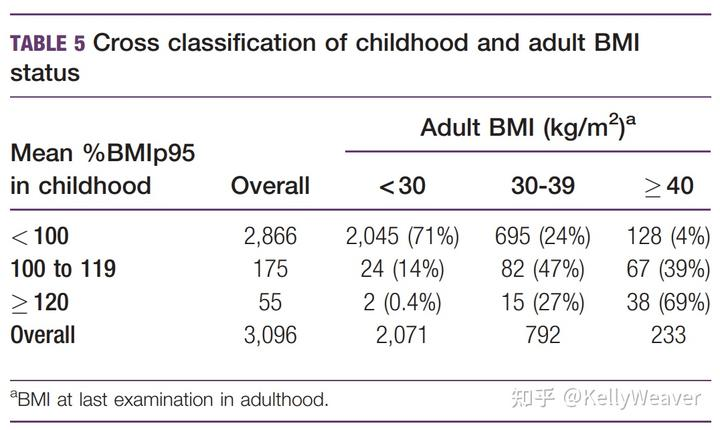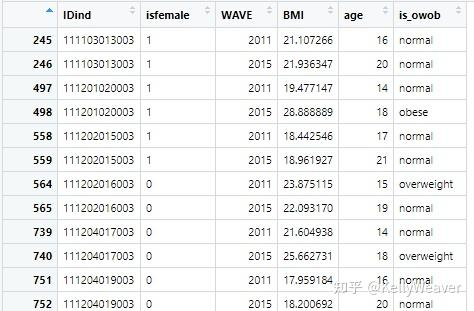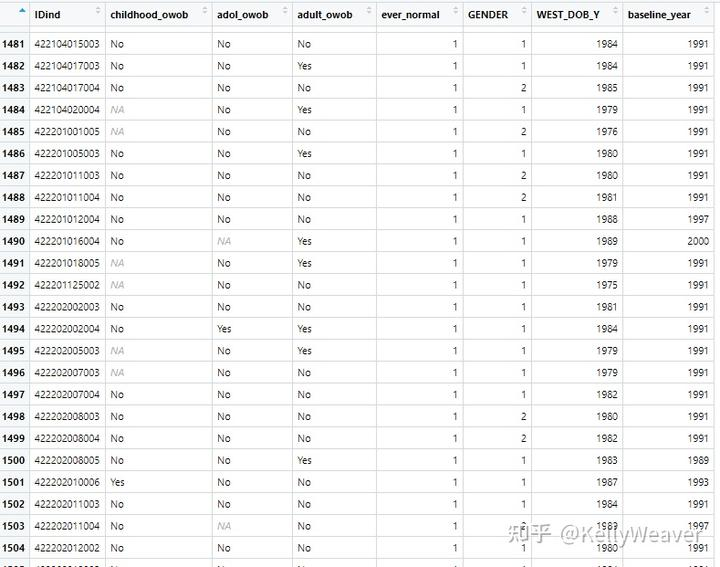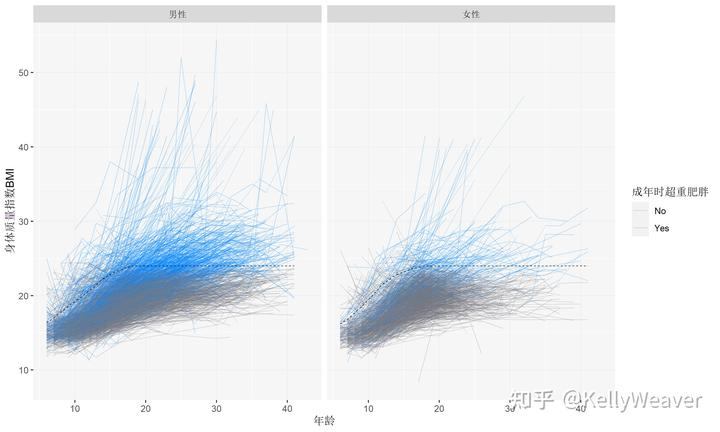# 从小就胖的人瘦下来的可能性大么？美国人群中的研究结果。这个表格，如果将 100 to 119 和≥120 的数据合并、将 30-39 和≥40 的数据合并得话，就能够算出：88% 的 BMI p95 大于 100 的儿童期肥胖者，都一直胖到了成年。

“从小就胖的人瘦下来的可能性大么”，这个问题里，有三个关键点：“从小就胖的人”、“（长大后）瘦下来”、“可能性”。

• 怎么才算“长大后瘦下来”？

• “从小就胖的人”是什么人？

“从小就胖”，这个词有两个部分：“从小”和“胖”。

• “可能性”是什么？

“6-17 岁的时候胖过，18 岁以后不再胖”，这看似轻描淡写的一句话，其实要求的是：要跟踪一个孩子长达最多 12 年，甚至以上。

• 暴露（自变量）1：6-11 岁时是否胖过
• 暴露（自变量）2：12-18 岁时是否胖过
• 结局（因变量）：18 岁以后是否胖过

``````# read the datasets
master=read.csv('mast_pub_12.csv')
master\$IDind=as.character(master\$Idind) # convert the IDs into character() type to make ease for viewing
master_short=master[,c('IDind','GENDER','WEST_DOB_Y')]
pexam=read.csv('pexam.csv')
pexam\$IDind=as.character(pexam\$IDind)

# merge the datasets
main=cbind(pexam,matrix(nrow = dim(pexam),ncol = 2))
names(main)[(dim(pexam)+1):(dim(pexam)+2)]=names(master_short)[2:3]
for (i in 1:dim(pexam)) {main[i,(dim(pexam)+1):(dim(pexam)+2)]=master_short[master_short\$IDind==pexam\$IDind[i],2:3]  }
length(unique(main\$IDind))

# remove observations without complete information (age, weight or height)
main\$age=main\$WAVE-main\$WEST_DOB_Y
main2=main[!is.na(main\$GENDER)&!is.na(main\$age)&!is.na(main\$WEIGHT)&!is.na(main\$HEIGHT),]
``````

``````# create marker variables in main2
main2\$has_x=main2\$age<18&main2\$age>=6
main2\$has_xy=main2\$age>=18&main2\$IDind%in%main2\$IDind[main2\$has_x]
# extract info of all eligible subjects
elig_ids=unique(main2\$IDind[main2\$has_xy])
elig_data=main2[main2\$IDind%in%elig_ids&main2\$age>=6,]
``````

``````# construct new variables
elig_data\$isfemale=factor(elig_data\$GENDER-1)
elig_data\$BMI=elig_data\$WEIGHT/(elig_data\$HEIGHT^2)*10000
## function for judging if a subject is overweight or obese
owob_criteria=read.csv('cut_points_for_childhood_obesity.csv')
is_owob=function(age,isfemale,BMI) {
isfemale=as.numeric(isfemale)-1
if (age>=18) {cutpoints=c(24,28)}  else {cutpoints=owob_criteria[owob_criteria\$age==age,c(2+2*isfemale,3+2*isfemale)]}
if (!is.na(BMI)) {
status='normal'
if (BMI>cutpoints) {status='overweight'}
if (BMI>cutpoints) {status='obese'}
}  else {status=NA}
return(status)
}
## apply the function
for (i in 1:length(elig_data\$IDind)) {elig_data\$is_owob[i]=is_owob(elig_data\$age[i],elig_data\$isfemale[i],elig_data\$BMI[i])}
``````“long format dataset”，不知道这个中文叫啥……

``````# construct new trunk dataset
d_wt=data.frame(elig_ids)
names(d_wt)='IDind'
# create new marker variables in individual-based dataset
## overweight and obesity
for (i in 1:length(d_wt\$IDind)) {
dataind=elig_data[elig_data\$IDind==d_wt\$IDind[i],]
### ever owob during [6,12)y
d_wt\$childhood_owob[i]=NA
if ('normal'%in%dataind\$is_owob[dataind\$age<12]) {d_wt\$childhood_owob[i]=0}
if ('obese'%in%dataind\$is_owob[dataind\$age<12]|'overweight'%in%dataind\$is_owob[dataind\$age<12]) {d_wt\$childhood_owob[i]=1}
### ever owob during [12,18)y
d_wt\$adol_owob[i]=NA
if ('normal'%in%dataind\$is_owob[dataind\$age>=12&dataind\$age<18]) {d_wt\$adol_owob[i]=0}
if ('obese'%in%dataind\$is_owob[dataind\$age>=12&dataind\$age<18]|'overweight'%in%dataind\$is_owob[dataind\$age>=12&dataind\$age<18]) {d_wt\$adol_owob[i]=1}
### ever owob after 18y
d_wt\$adult_owob[i]=NA
if ('normal'%in%dataind\$is_owob[dataind\$age>=18]) {d_wt\$adult_owob[i]=0}
if ('obese'%in%dataind\$is_owob[dataind\$age>=18]|'overweight'%in%dataind\$is_owob[dataind\$age>=18]) {d_wt\$adult_owob[i]=1}
}
d_wt\$childhood_owob=factor(d_wt\$childhood_owob,levels = c(0,1),labels = c('No','Yes'))
d_wt\$adol_owob=factor(d_wt\$adol_owob,levels = c(0,1),labels = c('No','Yes'))
d_wt\$adult_owob=factor(d_wt\$adult_owob,levels = c(0,1),labels = c('No','Yes'))
d_wt=left_join(d_wt,master_short)
d_wt=left_join(d_wt,unique(elig_data[,c('IDind','baseline_year')]))
``````前面筛过了一遍，到这还是有 NA，是因为这个人 6-11 岁或 12-17 岁期间的随访当中有一个不完整。后续的分析会分层分别分析，只要儿童期或者青春期有一个有数据，就可以作为这个层里面的有效样本点。

``````table1=cbind(data.frame(table(d_wt\$childhood_owob,d_wt\$adult_owob)),rep('childhood',4))
table2=cbind(data.frame(table(d_wt\$adol_owob,d_wt\$adult_owob)),rep('adolescence',4))
names(table1)=c('owob','adult_owob','freq','time')
names(table2)=c('owob','adult_owob','freq','time')
test=rbind(table1,table2)
test\$time=factor(test\$time,levels = c('childhood','adolescence'))

time_labs=c('12~17 岁时胖过','6~11 岁时胖过')
names(time_labs)=c('adolescence','childhood')
isfemale_labs=c('男性','女性')
names(isfemale_labs)=c('','')
breaks=c(0,0.2,0.4,0.6,0.8,1)

ggplot(data = test,mapping = aes(x=owob,y=freq,fill=adult_owob)) +  geom_bar(position = 'fill',stat = 'identity')+  scale_y_continuous(breaks = breaks, labels = scales::percent(breaks))+  facet_grid(.~time,labeller = labeller(time=time_labs))+  scale_fill_manual('成年后还胖',values = c("#0084ff","#bbbbbb"))+  scale_color_manual('成年后还胖',values = c("#0084ff","#bbbbbb"))+  theme(panel.background = element_rect(fill = '#f6f6f6'))+  labs(x='',y='占比')
ggsave(paste(getwd(),'/plots/barplot.png',sep = ''),width = 4.5,height = 4.5)
``````图中黑色虚线是超重 BMI 的判定线，虚线以上代表此时体重超重 / 肥胖

# 茉莉花新闻网

中国茉莉花革命网始创于2011年2月20日，受阿拉伯之春的感召，大家共同组织、发起了中国茉莉花革命。后由数名义工无偿坚持至今，并发展成为广受翻墙网民欢迎的新闻聚合网站并提供论坛服务。